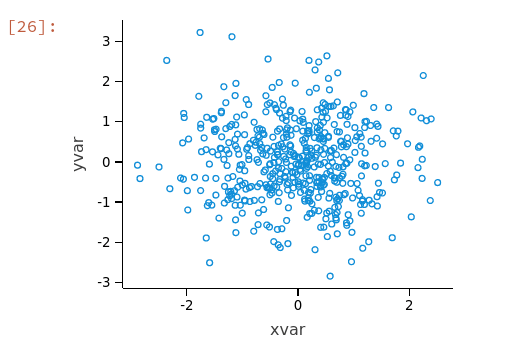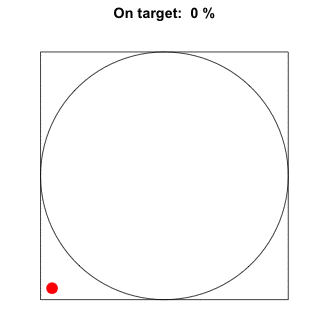### Sampling from Multivariate Distributions

Each sample is a vector of numbers instead of just one number.

How might taking into account multiple variables be useful?

Credit card companies often need to recognize fradulent credit card transactions so that their platforms remain secure, and customers are not charged for items they did not purchase. These companies may aim to preemptively detect when transactions are fraudulent, before they are reported.This could involve identifying certain factors: eg. rough location and time of where the transaction was initiated, merchant(s) the transaction was conducted and prior histories with the merchant.

Suppose that the factors tend towards a Gaussian distribution when a sufficiently large sample size is taken (CLT), anomalies which have a low probability of occurring would thus be more easily identified.

The various RNG methods as part of the s2 library which we will be using are detailed here

### Multinomial Distribution

Multinomial distributions are generalisations of the binomial distribution. While a binomial distribution can only predict two defined outcomes for each independent trial, such as success or failure, multinomial distributions are used when there can be more than 2 possible outcomes.

For instance, if there are 4 different blood types, A, B , O and AB. Let’s say that the blood distribution of a country has the following distribution:

 Blood Type A B O AB Percent (%) 29 24 39 8

Now, a hospital is short of blood and is tracking the patients carefully. What is the probability that of 10 patients walk in one after another whereby, 3 will be blood type A, 2 will be type B, 4 will be blood type O, and 1 type AB?

Let us try to “derive the PMF”, We first permutate (by taking into account all the possible trials for each variable,${10\choose4}*{10\choose3}*{10\choose2}*{10\choose1}= 12600$

We then multiply this value by their individual probabilities. Since we are checking if blood A will appear 3 times, type B 2 times and so on, we multiply them by the number of times they appear. We thus get:$12600*(P(blood A))^3*(P(blood B))^2*(P(blood O))^4*(P(blood AB))^1 = 0.0327\\=3.27\%$

The following code snippet puts 10,000 “patients” into different bins with the various proportion of bloodtypes as given earlier.

				
%use s2

//Array of doubles containing the blood type probabilities
var rvg = MultinomialRVG(100000, doubleArrayOf(0.257,0.241,0.089,0.406))
var bin = rvg.nextVector()

var total = 0.0

for (i in (0..bin.size-1)){
total += bin[i]
}

var bin0 = bin / total // bin0 percentage
var bin1 = bin / total // bin1 percentage
var bin2 = bin / total // bin1 percentage
var bin3 = bin / total // bin1 percentage

println(&quot;bin %% = %f, bin1 %% = %f, bin2 %% = %f&quot;.format(bin0, bin1, bin2, bin3))


				
bin % = 0.260480, bin1 % = 0.242890, bin2 % = 0.088220




Multivariate Normal Distributions

Understanding how the multivariate normal “Gaussian” distributions work

Recall for the uni variate Gaussian distribution, its shape is dictated by its mean and variance. A multivariate normal distribution i a vector in multiple normally distributed variables, such that any linear combination of variables is also normally distributed.

The mean for a multivariate Gaussian distribution is a column vector μ consisting of the mean (expectation) of each random variable. Meanwhile, the variance is known as a covariance.

### Recall: What is covariance?

Originally, variance measures the how much a randomly generated value differs from its probability distribution. However, we are now dealing with multi-variate distributions, which consist of multiple variables.

Covariance measures the fluctuation of two variables with respect to each other.
For covariance to have a large value, both variables have to have individually large variancesHow do we feature covariance as part of the normal distribution?

We make use of a covariance matrix$\sum$. The covariance matrix takes into account 2 dimensions, i and j. Thus, covariance matrix is$C_i,_j=\sigma(x_i,x_j)$

In a covariance matrix, the diagonal entries are the variances, and other entries are co-variances.$C = \begin{pmatrix}\sigma(x,x) & \sigma(x,y) \\\ \sigma(y,x) & \sigma(y,y) \end{pmatrix}$

To understand how the covariance works, let us first implement a base case, by plotting the a simple scatter plot of the multivariate normal “gaussian” distribution.
We will use the mean of the origin, and variance of 1.

				
%use s2, lets-plot

var mu = DenseVector(arrayOf(0.0, 0.0))

//covariance matrix
var sigma = DenseMatrix(arrayOf(
doubleArrayOf(1.0, 1.0),
doubleArrayOf(1.0, 1.0)))

var rvg = NormalRVG(mu, sigma)
rvg.seed(1234567890L)

// the number of data to generate
val N = 500
// generate the random data to plot
val data = mapOf&lt;String, List&gt;(
"xvar" to List(N) { i:Int-&gt; rvg.nextVector() },
"yvar" to List(N) { i:Int-&gt; rvg.nextVector()  }
)

// construct a scatter plot
val plot = ggplot(data) { x = "xvar"; y = "yvar" } + // supply data; set legends
ggsize(300, 250) + // plot size
geom_point(shape = 1) // draw a basic scatter plot
plotNote:

• doubleArrayOf() instead of DoubleArray as doubleArrayOf() allows us to specify the values, while DoubleArray() creates a one-dimensional array of doubles
• mapOf returns a read-only map with the specified contents, when it is given a list of pairs where the first value is the key and the second is the value.

This case would mean that x and y are independent (or uncorrelated). We can also double check this with the covariances of the samples given.

Now, we can also see how the co-variances change, by applying a linear transformation on the X and Y components of the matrix.

				

%use s2, lets-plot

var mu = DenseVector(arrayOf(0.0, 0.0))

//covariance matrix
var sigma = DenseMatrix(arrayOf(
doubleArrayOf(1.0, 1.0),
doubleArrayOf(1.0, 1.0)))

var rvg = NormalRVG(mu, sigma)
rvg.seed(1234567890L)

var transformation = DenseVector(arrayOf(5.0, 5.0))

// the number of data to generate
val N = 500
// generate the random data to plot
val rand_data = mapOf(&lt;String, List&gt;(
"yvar" to List(N) { i:Int&gt; rvg.nextVector() },
"xvar" to List(N) { i:Int&gt; transformation.multiply(DenseVector(arrayOf(
rvg.nextVector(),
rvg.nextVector()))) }
)

// construct a scatter plot
val plot = ggplot(rand_data) { x = "xvar"; y = "yvar" } + // supply data; set legends
geom_point(shape = 1) + // draw a basic scatter plot
coord_fixed()
plotNormal RVG can take on other parameters, however we will not go into much detail about them:

• mu
• the mean
• sigma
• the covariance matrix
• epsilon
• a precision parameter: when a number |x| ≤ ε,it is considered 0
• rnorm
• a random standard normal number generator

Apart from , there is also other ways of sampling random numbers – such as UniformDistributionOverBox and HyperSphere RVG – a High Dimensional Hypersphere

### Applications of the monte carlo method

We have previously learnt the idea behind monte-carlo sampling – that is being able to deduce a properties of a item by repeatedly sampling from it. In NM Dev, one way of implementing this idea is known as sampling from a high dimension box. Basically, we can dictate the box with 1D, 2D or 3D properties and place our object of interest- such as a circle inside the box. We can then make use of repeated sampling to deduce the properties. Let’s try it out!%use s2

//number of trials to sample
val N = 1000000

// create "box" of 1 m from origin in x and y direction
var rvg = UniformDistributionOverBox(RealInterval(-1.0, 1.0), RealInterval(-1.0, 1.0))

//counter to record values which fall within circle
var N0 = 0

for (i in (0..N-1)) {
var xy = rvg.nextVector()
//extract x and y coor of vector respectively
var x = xy
var y = xy
if (x * x + y * y &lt;= 1.0){
N0+=1
}

}
var pi = 4.0 * N0 / N

println("pi = %s".format(pi))



Note: The Real Interval  constructs a interval of “real” values from a lower to an upper bound. You can think of it as representing the number line!

				
pi = 3.1416